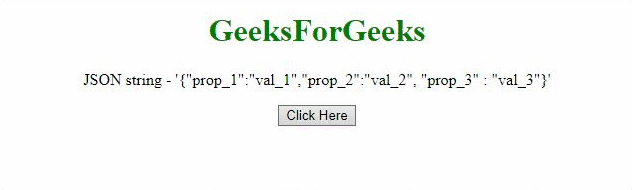# How to convert JSON string to array of JSON objects using JavaScript ?

• Difficulty Level : Easy
• Last Updated : 30 Jul, 2021

Given a JSON string and the task is to convert the JSON string to the array of JSON objects. This array contains the values of JavaScript object obtained from the JSON string with the help of JavaScript. There are two approaches to solve this problem which are discussed below:

Approach 1: First convert the JSON string to the JavaScript object using JSON.Parse() method and then take out the values of the object and push them into the array using push() method.

Hey geek! The constant emerging technologies in the world of web development always keeps the excitement for this subject through the roof. But before you tackle the big projects, we suggest you start by learning the basics. Kickstart your web development journey by learning JS concepts with our JavaScript Course. Now at it's lowest price ever!

• Example:
 ` ``<``html``> `` ` `<``head``> ``    ``<``title``> ``        ``How to convert JSON string to array``        ``of JSON objects using JavaScript?``    ```` `` ` `<``body` `style` `= ``"text-align:center;"``> ``     ` `    ``<``h1` `style` `= ``"color:green;"` `> ``        ``GeeksForGeeks ``    ````     ` `    ``<``p` `id` `= ``"GFG_UP"``>``     ` `    ``<``button` `onclick` `= ``"myGFG()"``>``        ``Click Here``    ````     ` `    ``<``p` `id` `= ``"GFG_DOWN"``>``     ` `    ``<``script``>``        ``var up = document.getElementById("GFG_UP");``         ` `        ``var JS_Obj = ``        ``'{"prop_1":"val_1", "prop_2":"val_2", "prop_3" : "val_3"}';``         ` `        ``up.innerHTML = "JSON string - '" + JS_Obj + "'";``         ` `        ``var down = document.getElementById("GFG_DOWN");``         ` `        ``function myGFG() {``            ``var obj = JSON.parse(JS_Obj);``            ``var res = [];``             ` `            ``for(var i in obj)``                ``res.push(obj[i]);``             ` `            ``down.innerHTML = "Array of values - ["``                            ``+ res + "]";``        ``}``    `` `` `` ` ``
• Output:Approach 2: This approach is also the same but using a different method. Convert the JSON string to the JavaScript object using eval() method and then take out the values of the object and push them to the array using push() method.

• Example:
 ` ``<``html``> `` ` `<``head``> ``    ``<``title``> ``        ``How to convert JSON string to array``        ``of JSON objects using JavaScript?``    ```` `` ` `<``body` `style` `= ``"text-align:center;"``> ``     ` `    ``<``h1` `style` `= ``"color:green;"` `> ``        ``GeeksForGeeks ``    ````     ` `    ``<``p` `id` `= ``"GFG_UP"``>``     ` `    ``<``button` `onclick` `= ``"myGFG()"``>``        ``Click Here``    ````     ` `    ``<``p` `id` `= ``"GFG_DOWN"``>``     ` `    ``<``script``>``        ``var up = document.getElementById("GFG_UP");``         ` `        ``var JS_Obj = ``        ``'{"prop_1":"val_1", "prop_2":"val_2", "prop_3" : "val_3"}';``         ` `        ``up.innerHTML = "JSON string - '" + JS_Obj + "'";``         ` `        ``var down = document.getElementById("GFG_DOWN");``         ` `        ``function myGFG() {``            ``var obj = eval('(' + JS_Obj + ')');``            ``var res = [];``             ` `            ``for(var i in obj)``                ``res.push(obj[i]);``             ` `            ``down.innerHTML = "Array of values - ["``                            ``+ res + "]";``        ``}``    `` `` `` ` ``
• Output:JavaScript is best known for web page development but it is also used in a variety of non-browser environments. You can learn JavaScript from the ground up by following this JavaScript Tutorial and JavaScript Examples.

HTML is the foundation of webpages, is used for webpage development by structuring websites and web apps.You can learn HTML from the ground up by following this HTML Tutorial and HTML Examples.

CSS is the foundation of webpages, is used for webpage development by styling websites and web apps.You can learn CSS from the ground up by following this CSS Tutorial and CSS Examples.

My Personal Notes arrow_drop_up